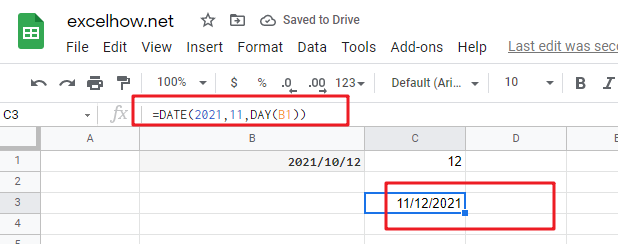It is important to adjust time periods when performing financial modeling. In Google Sheets, you can use the MONTH function to add a specific number of months to a date. The MONTH function takes one argument: the number of months you want to add. Here will show you how to add months to date in Google Sheets and even provide some examples that might be useful!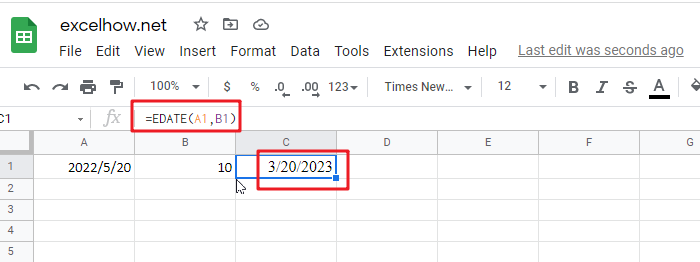## Method1: Add Months to Date using EDATE Function

Generic formula:

`=EDATE(Sart_date, months)`

### Arguments

• `Start_date`: the starting date on which you want to add months
• `Months`: the month that you wish to add

### Return Value:

The function will return the date that is the indicated number of months after the start_date. If you enter a negative number of months, it’ll count back from the end date.

When you enter the MONTH function, Google Sheets looks at the date in the cell that you specify as the start_date argument. It then adds the number of months that you entered as the second argument to that date. If you enter a negative number for the second argument, Google Sheets subtracts that number of months from the start_date.

To add a number of months is placed at B2 and add months to the date in cell A2. The formula for this would be:

`=EDATE(A1,B1)`Or simply enter the number you want in your formula, and it will be replaced with that value.

`=EDATE(A1,10)`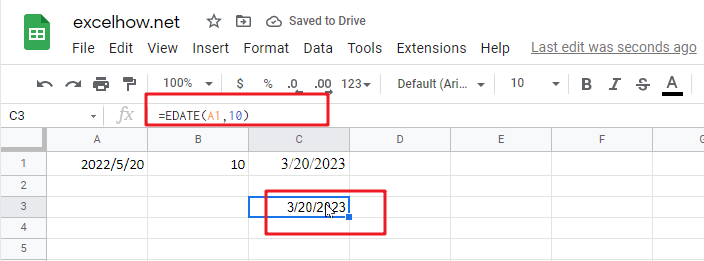### Explanation

This will add 10 months to the date in cell A1. The function will return the date that is the indicated number of months after the start_date. If you enter a negative number of months, it’ll count back from the end date.

The EDATE function is a great way to find out your next date. Simply enter any valid date and then select how many months away from now you want the new one! It’s really easy; just feed it with an amount less than or equal to the number of desired days between these two points-and voila.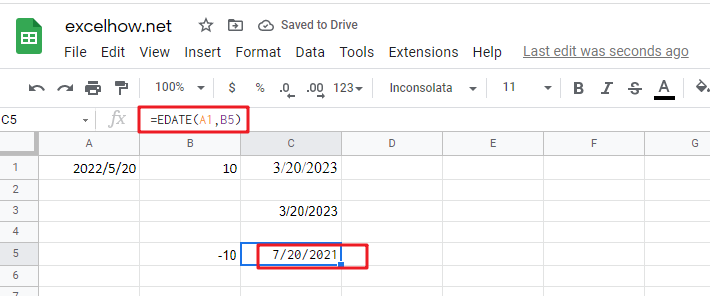## Method2: Add Months to Date using Date Function

Generic formula:

`=DATE(YEAR(date),MONTH(date))+months,DAY(date)))`

### Arguments

• `Date`: The starting date on which you want to add months.
• `Months`: Months are important when adding or subtracting a certain number of months from/to dates. They add the indicated amount, depending on whether it’s positive and negative values respectively; for example, -1 means one month less than what you want (in other words: ago).
• `YEAR`(date): The year component of the date in Date.
• MONTH(date): The month component of the date in Date.
• `DAY`(date): The day component of the date in Date.

### Return Value:

A date is the indicated number of months after the start_date. If you enter a negative number of months, it’ll count back from the end date.

### How This Formula Work

To add months to the date in cell A1, and a number of added is placed at B1. The formula for this would be as follows:

`=DATE(YEAR(A1),MONTH(A1)+B1,DAY(A1))`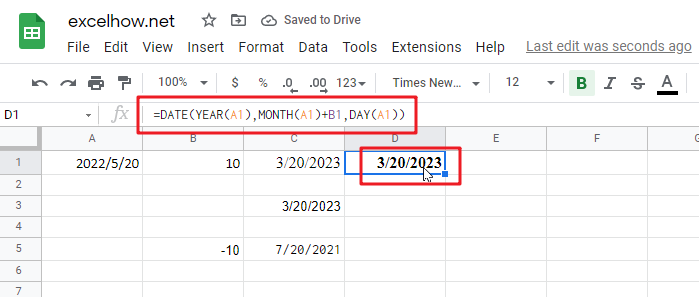With the Year, Month, and Day functions, you can easily create your date from any combination of these three values. With the Date function, you can create dates based on any year and month of your choice.

### Related Functions

The Google Sheets DAY function returns a day of a date (from 1 to 31).The DAY function is a build-in function in Microsoft Excel and it is categorized as a DATE and TIME Function.The syntax of the DAY function is as below:= DAY (date_value)…
The Google Sheets EDATE function returns the serial number that represents the date that is a specified number of months before or after a specified date.The syntax of the EDATE function is as below:=EDATE (start_date, months)…
The Google Sheets DATE function returns the serial number for a date.The syntax of the DATE function is as below:= DATE (year, month, day)…
The Google Sheets MONTH function returns the month of a date represented by a serial number. And the month is an integer number from 1 to 12.The syntax of the MONTH function is as below:=MONTH (serial_number)…
The Google Sheets YEAR function returns a four-digit year from a given date value, the year is returned as an integer ranging from 1900 to 9999. The syntax of the YEAR function is as below:=YEAR (serial_number)…

This post will guide you how to use Google Sheets TODAY function with syntax and examples.

## Description

The Google Sheets TODAY function get the current date as a date value. And it will be updated each time when your worksheet is changed or opened. This function does not need to add any arguments.

You can use the TODAY function to get the current date displayed on your worksheet. And it also can be used for calculating intervals. For example, if you want to know that person’s age (he was born in 1984), you can use the following formula:

=YEAR(TODAY())-1984

This formula will use the TODAY function as an argument for the YEAR function to get the current year, and then subtracts 1984, getting his age.

The TODAY function is a build-in function in Google Sheets and it is categorized as a Date function.

## Syntax

The syntax of the TODAY function is as below:

=TODAY()

There are no any arguments for the TODAY function, but you still need to use empty parentheses ().

Note:

• TODAY function will be updated on every edit made to the spreadsheet and it will impact spreadsheet performance.
• TODAY function will create a date without the current time, and if you wish to get the current date and time, and you can use the NOW function.
• TODAY function will always get the current date and the last date in the spreadsheet was recalculated, rather than remaining at the date when it was first entered.

## Google Sheets TODAY Function Examples

The below examples will show you how to use google sheets TODAY Function to return the current system date.

1# using the TODAY function to get the current date, enter the following formula in Cell B1.

`=TODAY()`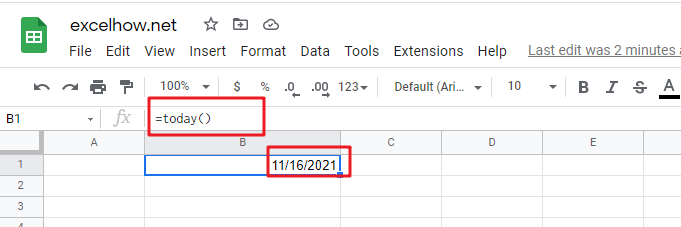2# Get the current date and then subtracts 10 days, using the following formula:

`=TODAY() -10`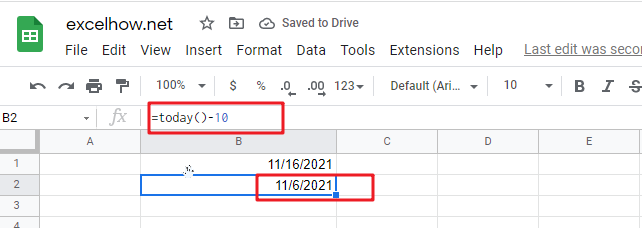3# Get the number of days between the current date and 03/14/2022, type the following formula:

`=DATEVALUE("03/14/2019")-TODAY()`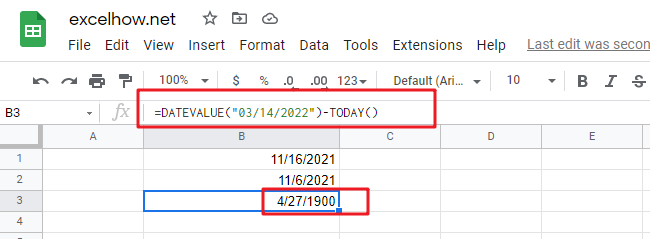4# Get the current day of the month, using the following formula:

`=DAY(TODAY())`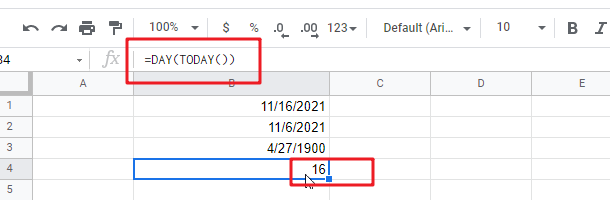5# Get the current month of the year, type the following formula in Cell B1:

`=MONTH(TODAY())`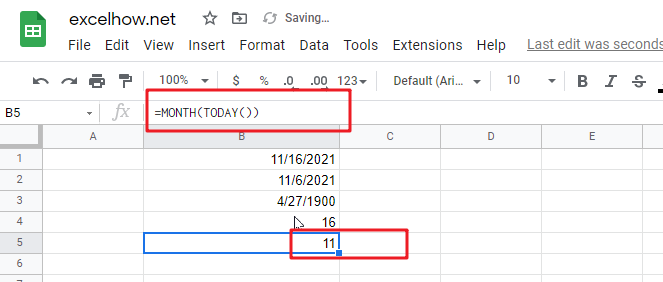This post will guide you how to use Google Sheets DAY function with syntax and examples.

## Description

The Google Sheets DAY returns a day of a date (from 1 to 31). You can use the DAY function to extract a day number from a given date in google sheets.

The DAY function can be used to get the day of the month as number between 1 to 31 from a given date. The purpose of this function is to get the day as number from a date and its returned values is a number indicating the day component in a date.

The DAY function is a build-in function in Google Sheets and it is categorized as a Date function.

## Syntax

The syntax of the DAY function is as below:

=DAY(date_value)

Where the DAY function arguments is:

• Date_text -This is a required argument. The date from which to extract the day. it can be a cell reference containing a date or a function returning a date type or a number.

Note:

• The date_value argument must be a valid date value, or it will return the #VALUE! Error.

## Google Sheets DAY Function Examples

The below examples will show you how to use google sheets DAY Function to return the day of a date.

#1 To extract the day of the month from a given date value in Cell B1, just using the following DAY function:

`=DAY(B1)`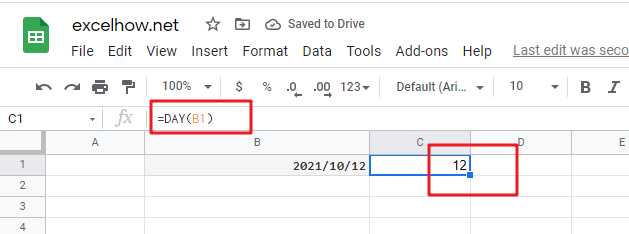Note:  The above excel formula will return the day of the date in Cell B1.

#2 the DAY function can be used to extract a day value and then passed the day value into another function called DATE function. Like below:

`=DATE(2021,11,DAY(B1))`